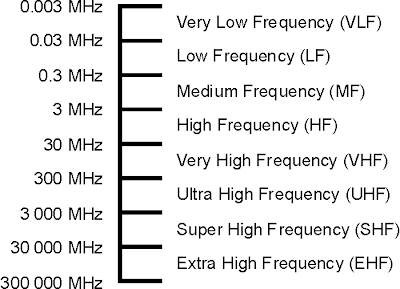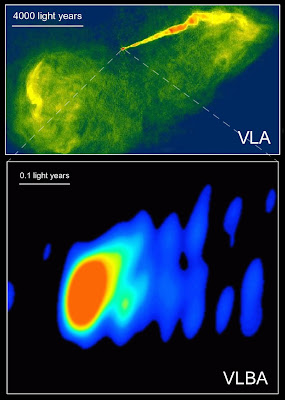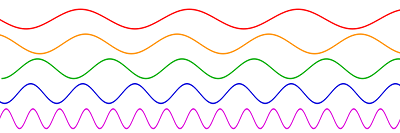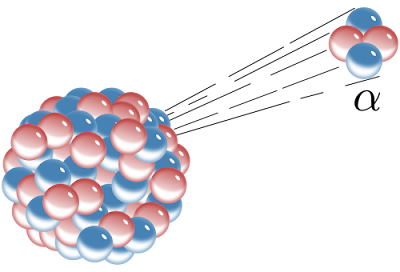## Monday, January 24, 2011

### Microwaves

Microwaves are a type of wave in the electromagnetic spectrum (see here). Their wavelengths are longer than any type of wave except for radio waves (see here).

The wavelength of a microwave can range from 1 millimeter (.001 meter) to 1 meter. This range is actually within the broader term radio wave, but on some scales, they are separate. Accordingly, the frequency range of microwaves is 300 MHz to 300 GHz (300,000,000 to 300,000,000,000 Hz).

Microwaves are specifically used in areas such as communication, power, and radar. Before fiber-optic cables were adopted into the phone system, microwaves were used for the same purpose. Radar, or the ability to map objects by bouncing waves off them, lies predominantly within the microwave region, but perhaps the most famous of microwave uses is the microwave oven. Microwave ovens bombard non-radioactive radiation into food, installing energy into, and therefore heating, it.The main component of the microwave oven is the magnetron. This is a cross-section of such a device which generates an electric field to produce microwaves. Magnetrons are generally for heating certain substances with microwaves, such as water, sugar, and fats in food, and sometimes, humans. Microwaves, however, are not specifically heat radiation.

Microwaves, just like all waves used for communication are divided into bands to organize their use. WIth microwaves, these bands are denoted by letter, (e.g. the L Band, ranging from 1 to 2 GHz) and each band has specific uses.

Yet another use of microwaves is in astronomy. There are many sources of microwaves in the heavens, but the most notable is the Cosmic Microwave Background Radiation. This radiation was emitted 379,000 years of the Big Bang, when the Universe became transparent due to the cessation of photon-plasma reactions. Plasma is ionized gas, and is therefore made of ionized atoms. When the temperature of the Universe had fallen enough to support complete atoms with electrons, the atoms became neutral, and the reactions stopped. The image of the Universe released at that moment was carried by gamma rays, but over time red shifting transferred these into microwaves, as they are today. More and less intense patches on the radiation indicate the density of different parts of the Universe. The differences were minute at the time, but gravity gradually increased the differences, and all of the structure in the large scale Universe was defined.An image adjusted from the original Cosmic Microwave Background to show temperature differences in the early Universe.

Microwaves have a variety of uses, from communication to heating to discovering the origins of the Universe.

Sources: http://en.wikipedia.org/wiki/Microwave, http://www.gallawa.com/microtech/mwave.html, http://en.wikipedia.org/wiki/Cosmic_microwave_background_radiation, etc.

## Sunday, January 16, 2011

### Radio Waves

Radio Waves are part of the electromagnetic spectrum (see here) and consist of photons in their wave form. Out of all of the electromagnetic waves, radio waves have the lowest frequency and highest wavelength.

The range of radio waves is approximately 3 Hz, meaning three wavelengths per second, to 300 GHz, or 300,000,000,000 Hz, although the boundary isn't clearly defined, and waves with frequencies even smaller than these exist. This range is equivalent to wavelengths from 1 millimeter (.001 meter) to 100,000 kilometers (100,000,000 meters).

Radio waves are both man-made, and occur from natural sources, and have many uses, due to the fact that most of the radio spectrum pass through Earth's atmosphere intact. The percentage of waves that pass through the atmosphere are shown on the picture below, along with the rest of the spectrum.Since radio waves can pass through the atmosphere, they are invaluable for communication purposes and information propagation takes place mainly in the radio spectrum. This portion of the spectrum is further divided into powers of 10. For example, the area from 3-30 Hz is known as Extremely Low Frequency (ELF), the area from 30-300 Hz is known as Super Low Frequency (SLF), the area from 300-3,000 Hz (3 kHz) is known as Ultra Low Frequency (ULF) and so on. The chart of frequencies above ULF are shown below.The first radio waves to be used for communication are AM radio waves, which extend from 148.5 kHz (148,500 Hz) to 30 MHz (30,000,000 Hz). These waves are in the Low, Medium, and High Frequency ranges. However, waves of these frequencies are produced naturally by the ionosphere and magnetic storms, causing interference. As a result, FM radio, falling entirely under the Very High Frequency range, is now used for long distance broad casts. This area of the spectrum is the busiest of all, as television, FM radio and mobile communications all resides within 30-300 MHz.

The very low frequencies, (VLF and lower) are used for military (submarine) communications as they are useful in subaquatic environments.

The higher frequencies also have uses. Super High Frequency (3-30 GHz, or 3,000,000,000-30,000,000,000 Hz) is used for radar, and Extremely High Frequency (30-300 GHz) is used for radio astronomy.The Very Large Array, located in New Mexico, USA, is used for radio astronomy. Large, parabolic satellite dishes concentrate the signals on a specific sources in the center of each individual telescope. Since many radio waves picked up from space have very long wavelengths, a very large apparatus is needed to capture a reasonable image. The Very Large Area has 27 radio telescope receivers, each 82 feet in diameter, and the images produced by each are compiled into a single one in the center.This is an optical (visible light) image of the M87 elliptical galaxy. The active center and jet of radiation from it are visible, but in visible light, most of the galaxy is a haze of stars.However, by use of radio astronomy, a much more specific picture can be taken. Unlike visible light, radio waves are not produced to a great extent by any part of the galaxy except the active center, and it can therefore be identified better in radio astronomy. The above image is taken by the Very Large Array (VLA). However, the bottom image, which is magnified tens of thousands of times more than the above one, is taken by the Very Long Baseline Array (VLBA). Since M87 is over 50 million light years away, a resolution of this magnitude can only be created by use of a planet sized radio telescope, which is essentially what VLBA is. Satellite dishes all over the world are synced together by a central computer in Europe, and therefore at least a small number of radio waves coming in over a Earth-sized area can be put together to get a very precise view, even if all the incoming waves aren't picked up.

Notable sources of radio waves from space include the Sun (only due to its proximity to Earth, because stars do not emit a majority of their energy in radio waves), galactic centers, such as the Milky Way itself, and active ones like M87, neutron stars, and very distant powerfully emitted objects such as quasars. The redshift effect of the quasars' movement away from us causes the waves to lengthen in wavelength as they approach Earth. As a result, many waves that start higher in frequency are radio waves when they reach Earth. This effect is most notable at very long distances, billions of light years away. Among objects at this distance, only quasars are bright enough to be visible, and are therefore excellent subjects of radio astronomy.

Sources: http://www.google.com/imgres?imgurl=http://www.electronics-radio.com/, http://en.wikipedia.org/wiki/Radio_astronomy, etc.

## Saturday, January 8, 2011

### Electromagnetic Spectrum

The Electromagnetic Spectrum, technically speaking, is the range of frequencies that a photon wave can assume. The photon is essentially the particle of light, and this particle has many interesting properties.

First, a photon has no mass, which means it can travel at a speed unlimited by its mass. The speed at which the light particle can travel in a vacuum defines the fastest known speed in the Universe, namely the speed of light, or 186,292 miles per second. Second, the photon can have properties of both a particle and a wave. But when dealing with the electromagnetic spectrum, the wave form of a photon is most important.

The electromagnetic spectrum deals with all possible photon waves, each of which are composed of the same particle, but have different energies. Along with energy, the three properties of a specific electromagnetic wave are amplitude, wavelength and frequency.Electromagnetic waves are in a sinusoid form, meaning that they are shaped like the red line above. The distance, A, from the sinusoid axis to the peak of the curve is known as the amplitude of the wave, and the distance between peaks is known as the wavelength, while the distance between crossings of the sinusoid axis are half of a wavelength. The remaining element, frequency, is how many wavelengths the wave goes through over a certain period of time. The horizontal axis (x) on the graph above is the passage of time, and over this time, the wave modulates, or moves from peak to peak, in a cyclic manner.The different electromagnetic waves are separated by varying amplitude and wavelength. The above chart shows five different wave types. The horizontal axis represents time, as before. On the top of the chart is the wave with the highest wavelength, but lowest frequency, and as one goes down the chart, the wavelength decreases and the frequency increases. The two properties are inversely proportional because an electromagnetic wave is traveling at a constant speed through a vacuum, the speed of light, and therefore the wavelength determines a length of time between wave peaks, namely frequency. Wavelength is measured in meters, while frequency is measured in a unit called a Hertz (Hz), with 1 Hz meaning one wavelength per second.The entire electromagnetic spectrum (click to enlarge), including names given to specific areas of the spectrum, some uses of particular wavelengths, and the corresponding wavelength and frequency axes. The plot is a log scale of base 10, meaning that instead of counting up 1, 2, 3... the graph counts 10^1, 10^2, 10^3... The frequency increases from the bottom to the top, starting at 10^6, and ending at 10^19, and the wavelength counts in meters (m), using symbols like cm to represent increments of meters.

The waves are as follows, from lowest to highest frequency are: radio waves, microwaves, infrared rays, visible light, ultraviolet rays, X-rays, and gamma rays. Each of these have their own individual posts. Click on one to enter and begin exploring the electromagnetic spectrum.

## Saturday, January 1, 2011

### Radioactive Decay

Radioactive Decay is the decomposition of an unstable atom into multiple parts. Often, this involves the emission of radiation, hence the name radioactive decay. Some elements of the periodic table have no stable atoms, while some have stable and unstable isotopes, meaning that the number of protons remains the same, but stability can vary with the number of neutrons.

There are 118 known elements on the periodic table, and, among these, numbers 43, 61, and all above 82 possess only isotopes that are radioactive. The remaining 80 have at least one neutron number that results in a stable nucleus, but a vast majority of the possibilities are radioactive, and overcome the force, known as the strong nuclear force or the strong interaction, that binds the particles in a nucleus together.

For example, the element oxygen has 8 protons, and the number of neutrons can range from 4 to 16, because it is known that there are physical limits on how many neutrons can exist for a certain number of protons, called the nuclear drip line. It is simply impossible for 3 neutrons and 8 protons to exist in a nucleus, and if these particles are shoved together, a proton will simply drip out. Similarly, it is impossible for 8 protons and 17 neutrons to be together in a nucleus, because a neutron will "drip" out. Therefore, these nuclei do not qualify as parts of atoms, as they are never in a whole state. Unlike these, isotopes of oxygen between 4 and 16 neutrons can exist. However, all but three of these are unstable, and decay to other atoms within seconds. Atoms with 8 protons and 8, 9, and 10 neutrons, corresponding to Oxygen-16, Oxygen-17, and Oxygen-18 are stable, and occur in nature.

Also, there are different ways that atoms can decay into others. The three most common were the first types of radiation isolated, and there were named alpha, beta, and gamma decay.

Alpha decay involves an unstable nucleus of an atom emitting an entire Helium-4 nucleus, that is, two protons and two neutrons, at once. This nucleus is also known as an alpha particle. The resulting nucleus of the parent atom has two less protons and two less neutrons than it did before alpha decay. Some argue that the Helium nucleus takes two electrons with it when an atom experiences alpha decay, because otherwise there would be an unbalanced charge, and some believe that two electrons are simply released into the environment. An example alpha decay reaction is pictured below.An example reaction involves Uranium-238, which, by alpha decay loses two neutrons and two protons. Because of this the resulting atom's atomic weight will drop from 238 to 234, and the atomic number will drop by two, becoming Thorium. Therefore the reaction is denoted

238,92U=234,92Th+4,2He

The notation above shows the two balanced sides of the reaction, with the Uranium-238 (first number before the atomic symbol is the entire weight, the second is the number of protons) atom on the left, and the Thorium-234 atom and the Helium nucleus (alpha particle) on the right. In this reaction the two elections are assumed to leave the atom with the Helium nucleus.

Beta decay involves a slightly more complicated chain of events, and there are two types: Beta negative and beta positive, denoted B- and B+ respectively. The B- reaction uses the weak nuclear force (one of the four fundamental forces of the Universe) to convert a neutron into a proton. However, there are some byproducts of this reaction, namely an electron and an electron antineutrino. Since a neutron is only slightly heavier than the proton, the loss of a tiny electron (and an electron antineutrino) is enough to convert it into a proton. Also, the change is mass is so minute that the atomic weight remains the same, but the atomic number goes up. This type of decay actually "increases" the complexity of the nucleus, instead of lowering it.This image is an example beta decay reaction. The main picture shows only the B- particle (electron) being emitted, while the inset shows the entire reaction. The neutron splits into three parts, of which the proton stays in the nucleus, the B- particle may leave the atom or stay and compensate for the gaining of a positive charge via the proton, and the tiny electron antineutrino is emitted. To fully understand this process, one must break it down into even smaller stages, by use of a Feynman Diagram.A Feynman Diagram graphically represents quantum phenomena to make them easier to understand. In this diagram, time progresses with respect to the vertical axis. The neutron, n, is shown as its three composite parts, u,d, and d, representing one up quark and two down quarks. The weak nuclear force has an effect on the final down quark only, instantly changing it to an up quark. As a result, the three new quarks are up, down, up (u,d,u) and these are the composite particles for a proton, hence the proton end product. However, to make this change happen, a W- boson, the carrier of the weak nuclear force, must be emitted from the down quark, to change it to an up quark. Since a down quark is heavier than an up one, the loss in mass again makes sense. The W- boson is very short lived, however, and nearly instantaneously splits into an electron and an electron antineutrino, denoted by the e- and ve+ respectively.

An example reaction of B- decay is the process of changing the Caesium-137 atom into the Barium-137 atom

137,55Cs = 137,56Ba + e- + ve+

Neutron-rich nuclei are more likely to undergo B- decay.

The other type of Beta decay is B+ decay. It is basically the opposite (in terms of particles) of the previous process, because a proton is converted into a neutron. Since the neutron is heavier than the proton, the reaction needs outside energy to add mass to the reaction (since energy can at any time by changed into mass and vice versa). This must be provided by the environment, and therefore this reaction cannot occur by itself in a vacuum. The byproducts of the reaction are the opposite of B- decay in charge; instead of a electron and an electron antineutrino being emitted, the positron (anti-electron) and electron neutrino are emitted. An example B+ decay reaction involving the transformation of Carbon-11 into Boron-11 is written below

11,6C = 11,5B + e+ + ve-

This reaction also involves the weak nuclear force, but with use of a W+ boson, rather than a W- one. The W+ boson is emitted when a up quark changes into a down quark. This particle then splits into the two mentioned above.

B+ decay is more likely to occur in proton-rich nuclei.

There are other rarer types of Beta decay, such as electron capture, where an electron is "captured" from the orbitals of the atom, and is pulled to the nucleus, where it combines with a proton to form a neutron and an electron antineutrino. An example reaction is

59,28Ni + e- = 59,27Co + ve+

Although the electron on the left side of this equation is presented as if it is separate from the atom, the electron actually originated from what was part of the atom, namely orbiting electrons.

There are also forms of Beta decay where the process happens twice simultaneously. These are called Double Beta Decay, and a similar double exists for electron capture.

Other simple types of radioactive decay include proton emission, if a nucleus is very rich in protons, and neutron emission, if a nucleus is very rich in protons. Note that this is different from proton and neutron "dripping" discussed earlier, because the nucleus does exist as one unit before the the proton or neutron is emitted.

Another famous type is the emission of a larger particle than an alpha particle, namely a heavier atomic nucleus. However, this type of decay, called cluster decay, only occurs among atoms that usually decay through the emission of an alpha particle. Some unstable atoms decay in different ways, with one occurring a certain percentage of the time, and another in the remaining percentage. An example is the atom Radium-223.

Usually, Radium-223 decays through alpha decay:

223,88Ra = 219,86Rn + 4,2He

but for one out of every one billion reactions, something else occurs, and the atom emits a Carbon-14 nucleus!

223,88Ra = 209,82Pb + 14,6C

The second reaction was the first of its kind known to occur and was discovered in 1984 at Oxford University. The heaviest known nucleus to be emitted in this fashion is Silicon-34, happening only once out of trillions and trillions of alpha decays from Plutonium-240, Americium-241, and Curium-242.

Finally, some atoms simply split into two atoms, through a process called spontaneous fission. This processes occurs when a neutron impacts the nucleus and splits it in two. Uranium, Plutonium, and Californium are three elements that have a chance for spontaneous fission, although they are more likely to decay through other processes. Californium-252 has a relatively high fission rate, with 3.09% of reactions result in fission. However, Uranium-235 decays through fission only seven reactions out of 100 billion! There are higher elements which predominately decay by fission, some of which are isotopes of Mendelevium and Rutherfordium. Some of these reactions emit neutrons in turn, and these can lead to chain reactions. Uranium-235 is one of these, and since each reaction gives out energy, it is one of the isotopes used in the detonation of atomic bombs.

Reactions of this type occur to all of the possible unstable isotopes. The chart that maps all the isotopes is known as the table of nuclides, linked to here.This is another version of the table of nuclides, which shows the predominant mode of decay for every known nuclide. The center of the band of nuclides are the most stable (those in black are stable), and they tend to get less stable away from the center. Isotopes that undergo B+ decay or Proton Emission occur on the right (proton-rich) side of the stable isotopes, while ones that undergo B- decay or Neutron Emission occur the left (neutron-rich) side of the stable isotopes. Also, alpha decay, cluster decay and fission tend to occur with heavier atoms, toward the upper right of the chart.

Some isotopes do not decay directly into a stable nucleus, and go through multiple steps of decay before reaching stability.An example is Uranium-238. Its decay chain is imaged above (click to enlarge), with each octagon containing the atomic number and mass of an isotope, while the arrows denote the decay chain, with letters representing the type of decay. The half-life, or average time to decay on each step, is also included under each octagon. Sometimes, the decay chain branches, when there are probabilities for other types of decay, but all of the branches converge on the stable isotope Lead-206.

Radioactive decay produces energy, and is therefore valuable as a potential power source, with drawbacks including lower feasibility and harmful radiation. Radiation is also used for other purposes, such as medical procedures, specifically for eliminating cancerous cells.

Sources: http://education.jlab.org/glossary/betadecay.gif, http://www.nature.com/nature/journal/v307/n5948/abs/307245a0.html, http://en.wikipedia.org/wiki/Table_of_nuclides_(complete)